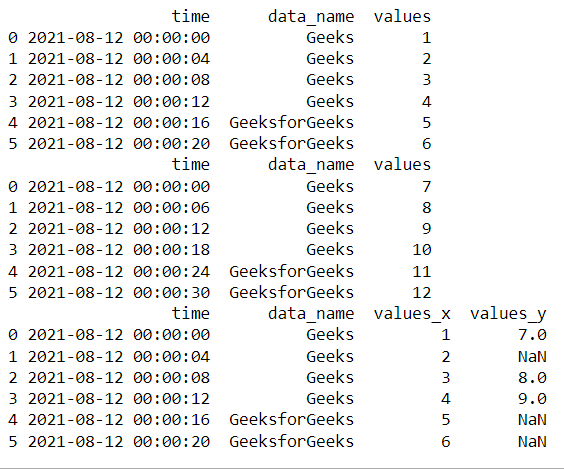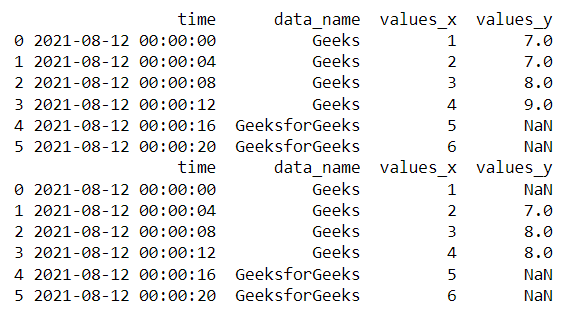Open in App
Not now

# How to Merge “Not Matching” Time Series with Pandas ?

• Last Updated : 19 Dec, 2021

In this article, we are going to see how to merge “Not Matching” Time Series with Pandas.

Time series is a sequence of observations recorded at regular time intervals. Time series analysis can be useful to see how a given asset, security, or economic variable changes over time

Usually, data consists of minute differences in values most likely in weather measurements or financial measurements, and when combining these time series dataframes the problem occurs in merging. pandas provide this amazing merge_asof  method to solve it. This helps in merging not matching timeseries data

## Merge “Not Matching” Time Series with Pandas

Assume we have two-time series dataframes df(left) and df1(right). when we are merging these two dataframes if the right dataframe value is not present in the left dataframe values then it is a problem right. so in this situation, we use the merge_asof which is included in pandas. It checks for the nearest previous values in right dataframe df1 and replaces it with that value

Syntax: pandas.merge_asof(left, right, on=None, left_on=None,  right_on=None, left_index=False, right_index=False, by=None, left_by=None, right_by=None, suffixes=(‘_x’, ‘_y’), tolerance=None,  allow_exact_matches=True, direction=’backward’)

## Python3

 `# importing packages ` `import` `pandas as pd ` ` `  `# creating dataframe df(left) ` `df ``=` `pd.DataFrame() ` ` `  `df[``'time'``] ``=` `pd.date_range(``'08/12/2021'``, ` `                           ``periods``=``6``, freq``=``'4S'``) ` ` `  ` `  `df[``'data_name'``] ``=` `[``"Geeks"``, ``"Geeks"``, ``"Geeks"``, ` `                   ``"Geeks"``, ``"GeeksforGeeks"``, ` `                   ``"GeeksforGeeks"``] ` `df[``'values'``] ``=` `[``1``, ``2``, ``3``, ``4``, ``5``, ``6``] ` ` `  ` `  `# creating datafrframe df1(right) ` `df1 ``=` `pd.DataFrame() ` ` `  `df1[``'time'``] ``=` `pd.date_range(``'08/12/2021'``, ` `                            ``periods``=``6``,  ` `                            ``freq``=``'6S'``) ` ` `  ` `  `df1[``'data_name'``] ``=` `[``"Geeks"``, ``"Geeks"``, ``"Geeks"``, ` `                    ``"Geeks"``, ``"GeeksforGeeks"``, ` `                    ``"GeeksforGeeks"``] ` `df1[``'values'``] ``=` `[``7``, ``8``, ``9``, ``10``, ``11``, ``12``] ` ` `  `# using merge_asof for merging left and right ` `df2 ``=` `pd.merge_asof(df, df1, on``=``'time'``, by``=``'data_name'``, ` `                    ``tolerance``=``pd.Timedelta(``'2s'``)) ` ` `  `# view data ` `print``(df) ` `print``(df1) ` `print``(df2) `

Output:We merge these dataframes (df and df1) on “time” by “data_name” column but some time values are not matching. For example, in the second row, time in A is two seconds behind the time in B.

### Another question is how the values_y is generated?

In the first row the time matches in both dataframes df and df1 so the values are the same and In the second-row df has 4s whereas df1 has 6s both are not equal so this merge_asof looks in the right dataframe df1 like which is the previous nearest value so here 0s is the nearest but why the value is NaN because we mention the tolerance timeframe to look is 2s but it is 6s so the value_y in 00:00:004 is NaN

Merge_asof also provides an option to exclude exact matches (attr=allow_exact_matches).

1. If True, allow matching with the same ‘on’ value (i.e. less-than-or-equal-to / greater-than-or-equal-to)
2. If False, don’t match the same ‘on’ value (i.e., strictly less-than / strictly greater-than).

## Python3

 `# importing packages ` `import` `pandas as pd ` ` `  `# creating dataframe ` `df ``=` `pd.DataFrame() ` ` `  `df[``'time'``] ``=` `pd.date_range(``'08/12/2021'``,  ` `                           ``periods``=``6``, ` `                           ``freq``=``'4S'``) ` ` `  ` `  `df[``'data_name'``] ``=` `[``"Geeks"``, ``"Geeks"``, ``"Geeks"``, ` `                   ``"Geeks"``, ``"GeeksforGeeks"``, ` `                   ``"GeeksforGeeks"``] ` `df[``'values'``] ``=` `[``1``, ``2``, ``3``, ``4``, ``5``, ``6``] ` `# creating dataframe ` `df1 ``=` `pd.DataFrame() ` ` `  `df1[``'time'``] ``=` `pd.date_range(``'08/12/2021'``,  ` `                            ``periods``=``6``, freq``=``'6S'``) ` ` `  ` `  `df1[``'data_name'``] ``=` `[``"Geeks"``, ``"Geeks"``, ``"Geeks"``, ` `                    ``"Geeks"``, ``"GeeksforGeeks"``,  ` `                    ``"GeeksforGeeks"``] ` `df1[``'values'``] ``=` `[``7``, ``8``, ``9``, ``10``, ``11``, ``12``] ` ` `  `# allow_exact_matches=True for merging ` `df3 ``=` `pd.merge_asof(df, df1, on``=``'time'``, ` `                    ``by``=``'data_name'``, ` `                    ``allow_exact_matches``=``True``) ` `# view data ` `print``(df3) ` `# allow_exact_matches=False for merging df and df1 ` `df4 ``=` `pd.merge_asof(df, df1, on``=``'time'``,  ` `                    ``by``=``'data_name'``, ` `                    ``allow_exact_matches``=``False``) ` `# view data ` `print``(df4) `

Output:My Personal Notes arrow_drop_up
Related Articles